# Logic Gates

### Logic gates and Truth Tables StarterYou are stranded on an Island when you bump in to 3 people. Of the three people (Alice, Bill, Charlie):

• One is a knight
• One is a knave
• One is a spy

On this island:

• Knights always tell the truth
• Knaves always lie
• Spies can do either

Alice says: “Charlie is a knave.

Bill says: “Alice is a knight.”

Charlie says: “I am the spy.

Who is the knight, who the knave, and who the spy?

### Boolean Logic

All data stored in computer systems is stored in binary format, which means that any calculations that are made must be done using only True and False values, this is known as Boolean logic.

Take for the instance the set up below: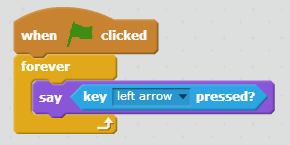When we run the code above, scratch will either say “True” or “False”, depending on whether you press the let arrow key.

### Truth Tables

When we are working out what possibilities exist for a particular Boolean logic structure, we can use a truth table to represent that data.

Using the previous example we can produce a simple truth table for the results.

 Input Output 1 1 0 0

AND Gates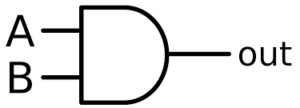A Boolean AND gate works by the following rules:

1. If both inputs are true, then the output will be True.
2. Otherwise the output will be false

Scratch Example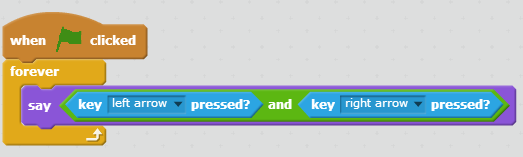Challenge 1 – AND Gate truth table

Can you fill out the truth table for an AND gate?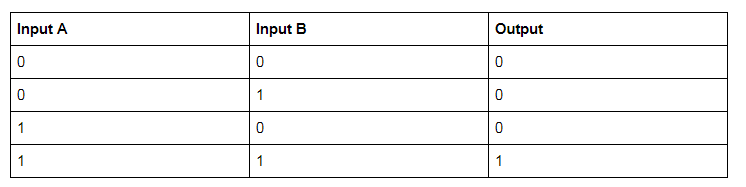OR Gates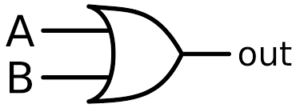A Boolean OR gate works by the following logic:

1. If either input is True, the output is True.
2. If both inputs are True, the output is True.
3. Otherwise the output is false.

Scratch Example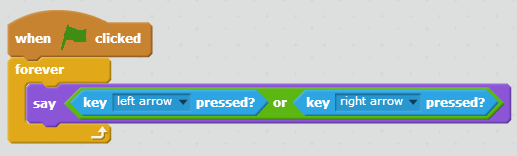Challenge 2 – Can you work out the truth table for a Boolean OR gate?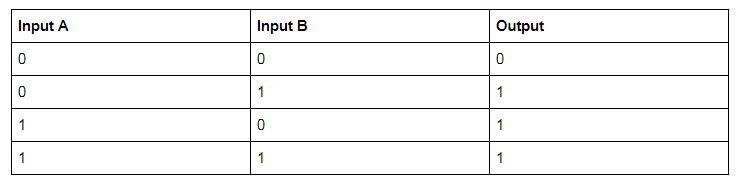### NOT Gate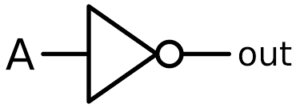A Boolean NOT gate simply reverses whatever input is given.

1. If the input is True, the output is False
2. If the input is False, the output is True

Scratch Not gate Example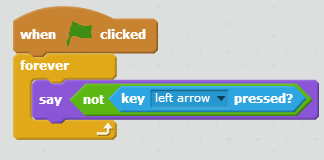Challenge 3 – Can you write a truth table for a NOT gate?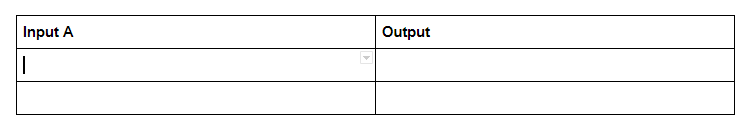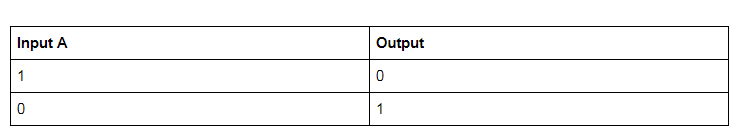Have a go at connecting up multiple logic gates to see what happens, using the simulator below. Can you work out the inputs and outputs for the following examples.

Example 1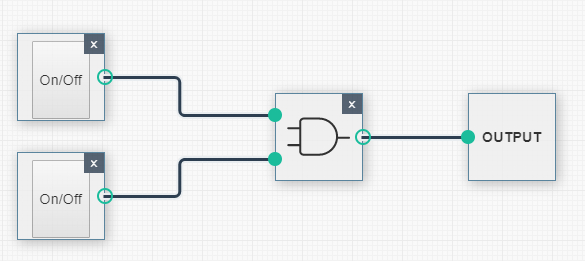Example 2Example 3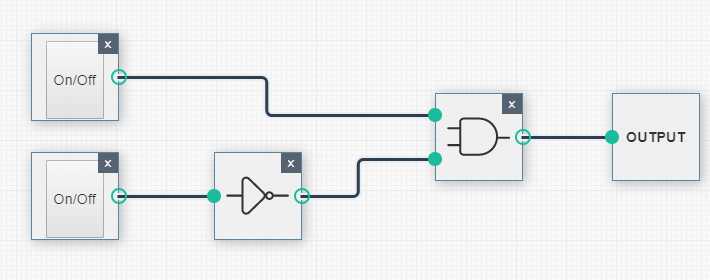Example 4Activity 2 – Applying Logic Gates

Using the link below, see if you can make the following systems:

Logic Gates Application Simulator

System 1 – Fan cooling system.

If the main system switch is turned on, then the fan will operate when:

• Every 5 seconds
• When The manual override button is pressed.

System 2 – Basic Alarm System

Make a system where if any of the 3 sensors (use 3 switches to represent sensors) are triggered, the alarm light will flash RED.

System 3 – Advanced Alarm System